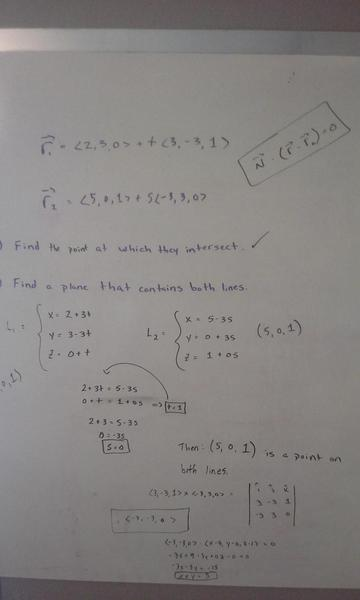# Consider the lines & find an equation of a plane [Cal III]

• BoonBoon
Yes, you are absolutely right. Having 0 as the coefficient of Z is what scared me. My professor hadn't covered what that meant very well, so I was confused. Thank you very much for your input.

#### BoonBoon

Consider the Lines Below:

r
= <2, 3, 0> + t<3, -3, 1>

r = <5, 0, 1> + s<-3, 3, 0>

A.) Find the point at which the given lines intersect.
B.) Find an equation of the plane that contains these lines.

## Homework Equations

[/B]
The vector equation of a line is given by r = r0 - tv

The equation of a plane is given by N . (r - r0)

## The Attempt at a Solution

I worked everything to the best of my ability but I ended up getting an equation of a line as opposed to the equation of a plane... Did I do something wrong? Here is a picture of my work:BoonBoon said:
Consider the Lines Below:

r
= <2, 3, 0> + t<3, -3, 1>

r = <5, 0, 1> + s<-3, 3, 0>

A.) Find the point at which the given lines intersect.
B.) Find an equation of the plane that contains these lines.

## Homework Equations

[/B]
The vector equation of a line is given by r = r0 - tv

The equation of a plane is given by N . (r - r0)

## The Attempt at a Solution

I worked everything to the best of my ability but I ended up getting an equation of a line as opposed to the equation of a plane... Did I do something wrong? Here is a picture of my work:I don't see anything wrong with your work. The two lines intersect at (5, 0, 1) (I checked), and the equation of the plane is indeed x + y = 5. In three dimensions this is a plane.

Mark44 said:
I don't see anything wrong with your work. The two lines intersect at (5, 0, 1) (I checked), and the equation of the plane is indeed x + y = 5. In three dimensions this is a plane.

Thank you so much. I think I figured out the error in my way of thinking. For some reason I was thinking of my answer as looking like a cube in R3. But since x + y = 5 is a line in R2, I could just scale the Z value infinitely in either direction in R3, as long as I'm restricted to that line. Thus, I would have a plane. Interesting. Thanks so much for your response!

You say " I ended up getting an equation of a line" but that is not true. A line cannot be written as a single equation in 3 dimensions. What you have is a plane in which the coefficient of z happens to be 0. That simply means that the plane is parallel to the z-axis. You can think of it as the line defined by x+ y= 5 in the xy-plane extended parallel to the z-axis.

HallsofIvy said:
You say " I ended up getting an equation of a line" but that is not true. A line cannot be written as a single equation in 3 dimensions. What you have is a plane in which the coefficient of z happens to be 0. That simply means that the plane is parallel to the z-axis. You can think of it as the line defined by x+ y= 5 in the xy-plane extended parallel to the z-axis.

Yes, you are absolutely right. Having 0 as the coefficient of Z is what scared me. My professor hadn't covered what that meant very well, so I was confused. Thank you very much for your input.

Last edited: